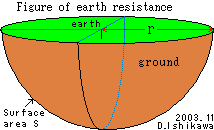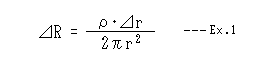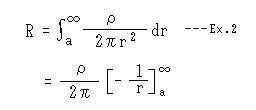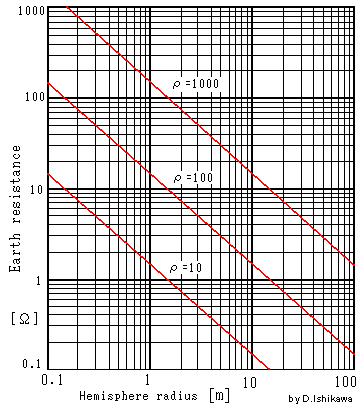﻿

# Calculation of ground resistance and concept of ground resistance

The actual grounding resistance can not be easily calculated. However, the grounding resistance wanted to know whether there is any nature. To assume a uniform soil, I tried to calculate the required ground resistance. Since this method is my own thought, I think it may be wrong somewhere. Please tell me if any errors.

## Calculation of ground resistance

Grounding resistance, is the resistance from the earth electrode to depths of the earth. Determined by calculating the resistivity of the soil from the ground resistance, grounding resistance and let's see what belongs to nature. The resistivity of the soil is about 10 - 1000 [Ω m], the normal soil is about 100 [Ω m].

### Diffusion of earth current to the groundThe earth is given to a hemisphere center with pattern diagrams of a left earth OHM meter calculation, and respect on the hemisphere is made a ground level.

The current that flows from the earth of the center diffuses to the earth in all directions when assuming the one with uniform anywhere electrical nature of the soil, and the point on the hemisphere is thought to be a flow of an equal anywhere current. Therefore, the point on the hemisphere is thought that potential is all equal. There is not an influence electrically even if the point where potential is equal is connected with the lead mutually either. Therefore, you may think this hemisphere surface to be a thin conductor.

This hemisphere radius It is the surface area when it is assumed r [m] Then, the surface area S [m2] is by half of the ball

S = 2*π*r2

### Calculation of ground resistance by integration

Minute thickness in this hemisphere surface Δr The soil has adhered. This thickness Δr Resistance only of this soil ΔR [Ω] This, the resistivity of the soil ρ [Ω*m]

ΔR = ρ* Δr/SIt becomes like expression 1 in a word. The ground resistance becomes it from the range of this expression 1 where it is when having added to infinity. That is, it will only have to integrate expression 1.If the radius integrates the resistance of the soil of minute thickness that adheres to this hemisphere from an infinite smallness to infinity, the ground resistance becomes infinity.

Therefore, this hemisphere radius is limited It decided to integrate from a [m] to infinity. It is computable like expression 2.

This result

R = ρ/(2*π*a) - - expression 3.

### Numerical result of ground resistance in domed earth poleA left graph is a calculation of the ground resistance in a domed earth pole by expression 3.

From this graph to ρ=100[Ω*m] level of unquestionable in the following resistance of hemisphere radius 5m-10m or more even if it becomes 1.6 Ω-3 Ω or less and it disregards itIn a word, the ground resistance is to decide in the state within 5-10m in radius. (50-100m or more in radius in ρ=1000[Ω*m])

It is enlarged that it can be only a ground electrode (earth electrode) or when the ground electrode surrounding is made a material that electrifies it easily, it is possible to lower the ground resistance.

The ground resistance is to depend on the radius resistivity of the soil in the hemisphere within about 10m and the size of the earth pole greatly.

The size of the earth pole to do can be presumed to the value of a necessary ground resistance from the graph ..on... For instance, to obtain the ground resistance below 100 Ω, it is understood to only have to make the one more than the equal to the hemisphere of 1.5m in radius an earth pole in ρ=1000 [Ω*m]. In a word, it is understood to have the input of the ground bar of 1.5m or more in length within the range of 3m or more in diameter how many it is.

## Method of reducing ground resistance

• The electrode of the earth is enlarged as much as possible.
• The electrode of the earth is buried as deeply as possible.
• These are connected in parallel mutually by setting up two or more earth in a place away from the electrode of the earth by ten meters or more.
• Charcoal, the salt, and the earth resistance decrease medicine that conducts electricity easily to the soil in the vicinity of earth electrode The resistivity of the soil is lowered mixing (brand name Athlon, San-earth, [Doudenkurito], [Chikogeru], [Amoru-earth], and [Nippi-earth], etc.).
• The ground resistance can be reduced further if these methods are combined and it does.

As for the earth electrode, to reduce the ground resistance when easily summarizing it, it is understood that setting it up so that it to be easy conduct electricity to the large range large, and as deeply as possible as much as possible as much as possible is important.